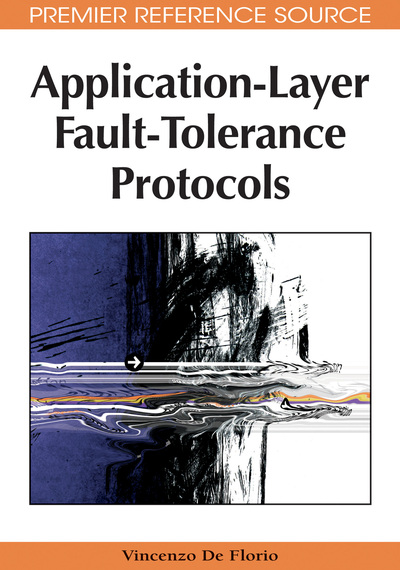# Measuring and Assessing Tools

Vincenzo De Florio (PATS Research Group, University of Antwerp and iMinds, Belgium)
DOI: 10.4018/978-1-60566-182-7.ch010
Available
\$37.50
No Current Special Offers

## Abstract

As mentioned in Chapter I, a service’s dependability must be justified in a quantitative way and proved through extensive on-field testing and fault injection, verification and validation techniques, simulation, source-code instrumentation, monitoring, and debugging. An exhaustive treatment of all these techniques falls outside the scope of this book, nevertheless the author feels important to include in this text an analysis of the effect on dependability of some of the methods that have been introduced in previous chapters.
Chapter Preview
Top

## Reliability Analysis Of The Tiran Distributed Voting Mechanism

As mentioned in Chapter 9, a number of applications are structured in such a way as to be straightforwardly embedded in a fault-tolerance architecture based on redundancy and consensus. Applications belonging to this class are, for instance, parallel airborne and spaceborne applications. The TIRAN Distributed Voting mechanism provides application-level support to these applications. This section analyses the effect on reliability of the enhancements to the TIRAN Distributed Voting mechanism described in the mentioned chapter, that is, management of spares, dealt with in Sect. 2.1, and fault-specific recovery strategies supported by the α-count feature, analyzed in Sect. 2.2.

### Using ARIEL to Manage Spares

This section analyses the influence of one of the features offered by ARIEL—its ability to manage spare modules in N-modular redundant systems—that has been introduced and discussed in Chapter 6 and Chapter 9.

Reliability can be greatly improved by this technique. Let us first consider the following equation:R(0)(t) = 3R(t)2 - 2R(t)3, (1) i.e., the equation expressing the reliability of a TMR system with no spares, R(t) being the reliability of a single, non-replicated (simplex) component. Equation (1) can be derived for instance via Markovian reliability modeling under the assumption of independence between the occurrence of faults (Johnson, 1989).

With the same technique and under the same hypothesis it is possible to show that, even in the case of non-perfect error detection coverage, this equation can be considerably improved by adding one spare. This is the equation resulting from the Markov model in Figure 1, expressed as a function of error recovery coverage (C, defined as the probability associated with the process of identifying the failed module out of those available and being able to switch in the spare (Johnson, 1989)) and time (t):R(1)(C, t) = (-3C2 + 6C) × [R(t) (1 - R(t))]2 + R(0)(t). (2)

Figure 1.

Markov reliability model for a TMR-and-1-spare system. λ is the failure rate, C is the error recovery coverage factor. A “fail safe” state is reached when the system is no more able to correctly perform its function, though the problem has been safely detected and handled properly. In ‘Fail unsafe,’ on the contrary, the system is incorrect, though the problem has not been handled or detected. Every other state is labeled with three digits, d1d2d3, such that d1 is the number of non-faulty modules in the TMR system, d2 is the number of non-faulty spares (in this case, 0 or 1), and d3 is the number of undetected, faulty modules. The initial state, 310, has been highlighted. This model is solved by Eq. (2). ©1998 IEE. Used with permission.

Appendix A gives some mathematical details on Eq. (2).

Adding more spares obviously implies further improving reliability. In general, for any N≥ 3, it is possible to consider a class of monotonically increasing reliability functions,

`(R(M)(C, t))M>0,(3)`

corresponding to systems adopting N+ M replicas. Depending both on cost and reliability requirements, the user can choose the most-suited values for M and N.

Note how quantity (2) is always greater than quantity (1) as R(0)(t) and (-3C2 + 6C) are always positive for 0< C≤ 1. Figure 2 compares Eq. (1) and (2) in the general case while Figure 3 covers the case of perfect coverage. In the latter case, the reliability of a single, non-redundant (simplex) system is also portrayed. Note furthermore how the crosspoint between the three-and-one-spare system and the non-redundant system is considerably lower than the crosspoint between the latter and the TMR system—R(t) ≈ 0.2324 vs. R(t) = 0.5.

The reliability of the system can therefore be increased from the one of a pure NMR system to that of N-and-M-spare systems (see Figure 2 and Figure 3).

## Complete Chapter List

Search this Book:
Reset